# 7.2.3 Geometrical Constructions, PT3 Focus Practice

Question 6:
Diagram below in the answer space shows part of a triangle ABC.

(a)Solution:
(a)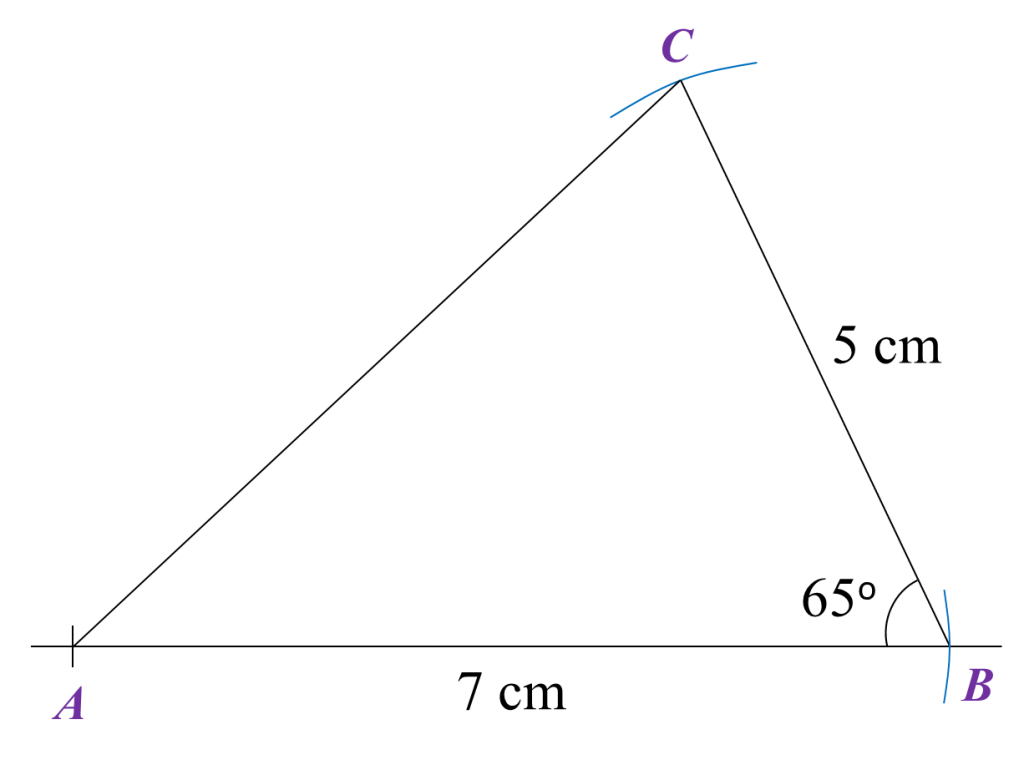(b)
$\angle ACB={72}^{o}$

Question 7:
Diagram below shows a quadrilateral ABQR.(a) Using only a ruler and a pair of compasses, construct the diagram using the measurement given.
Begin from the straight lines AB and BQ provided in the answer space.
(b) Based on the diagram constructed in (a), measure the length, in cm, of QR.

(a)Solution:
(a)(b)
QR = 5.9 cm

# 7.2.2 Geometrical Constructions, PT3 Focus Practice

7.2.2 Geometrical Constructions, PT3 Focus Practice 2

Question 4:
Diagram below shows a parallelogram PQRS. The point T lies on PQ such that ST is perpendicular to PQ.Using only a ruler and a pair of compasses, construct parallelogram PQRS, beginning from the lines PQ and PS provided in the answer space.

: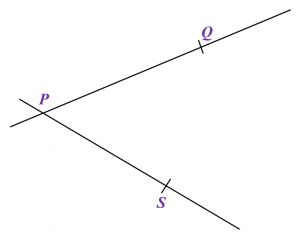Solution
:Question 5:
Diagram below shows a triangle ABC.(a) Using only a ruler and a pair of compasses, construct triangle ABC, beginning with the straight line BC in the answer space.
(b)   Hence, measure the length, in cm, of BA.

:
(a)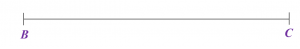Solution
:
(a)(b)
BA = 4.5 cm

# 7.2.1 Geometrical Constructions, PT3 Focus Practice

7.2.1 Geometrical Constructions, PT3 Focus Practice
Question 1:
Diagram below in the answer space shows part of triangle ABC.
(a) Using a pair of compasses, a protractor and a ruler, draw a triangle ABC starting from the line given in the answer space with AB = 4.5 cm, BC = 6 cm and ∠ABC = 105o.
(b) Measure ∠BCA.

:
(a)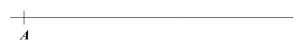Solution
:
(a)(b)
∠BCA = 31o

Question 2:
Diagram below shows a triangle ABC drawn not to scale.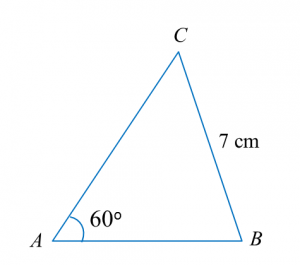Diagram in the answer space shows a straight line AB.
(a) Using only a ruler and a pair of compasses, construct
(i) triangle ABC to the measurements shown in diagram above,
(ii)   the perpendicular line to the straight line AC which passes through the point B.
(b)   Measure the perpendicular distance between straight line AC and point B.

:
(a)(i),(ii)Solution
:
(a)(i),(ii)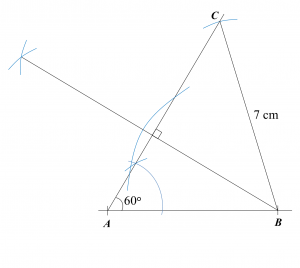(b)
5.3 cm

Question 3:
Diagram below shows a pentagon ABCDE.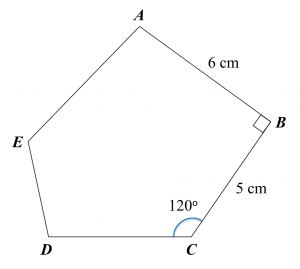Using only a ruler and a pair of compasses, construct the diagram, beginning from the straight lines CD and DE provided in the answer space.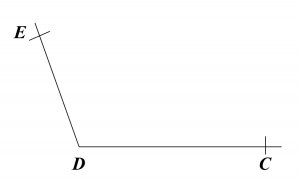Solution: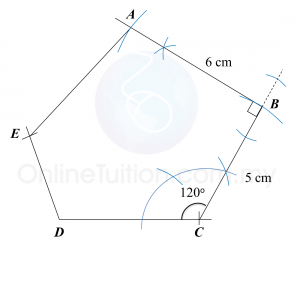# 5.4.3 Extraction of Metals from their Ores Using Coke

5.4.3 Extraction of Metals from their Ores Using Coke

1. In industries, metal ores which are less reactive than carbon are heated with carbon to obtain pure metal.
2. Pure metals which can be extracted using carbon are zinc, iron, tin and lead.

Extraction of Tin from its OreExtracting tin ore in a blast furnace

1. Tin ore exists naturally as cassiterite (or tin oxide).
2. Tin ore is washed with water to remove sand, clay and others impurities.
3. After that, tin ore is roasted to take away foreign matter such as carbon, sulphur and oil.
4. Lastly, the tin ore is mixed with carbon and limestone in the form of coal and is heated in a blast furnace at a high temperature.
5. The function of the limestone is to remove impurities.
6. Reduction reaction occurs during heating, carbon which is more reactive that tin removes oxygen from the tin oxide to produce pure tin and carbon dioxide.7.
Pure tin flows out from the furnace into moulds to harden as tin ingots.
8. At the same time, the limestone (calcium carbonate) breaks down to form quicklime (calcium oxide) which reacts with impurities to form slag

# 6.2.2 Pythagoras’ Theorem, PT3 Focus Practice

Question 6:
In diagram below, ABCH is a square and DEFG is a rectangle. HCDE is a straight line and HC = CD.Find the perimeter, in cm, of the whole diagram.

Solution:

Question 7:
In the diagram, ABC and EFD are right-angled triangles.Calculate the perimeter, in cm, of the shaded region.

Solution:

Question 8:
In the diagram, ABD and BCE are right-angled triangles. ABC and DBE are straight lines. The length of AB is twice the length of BC.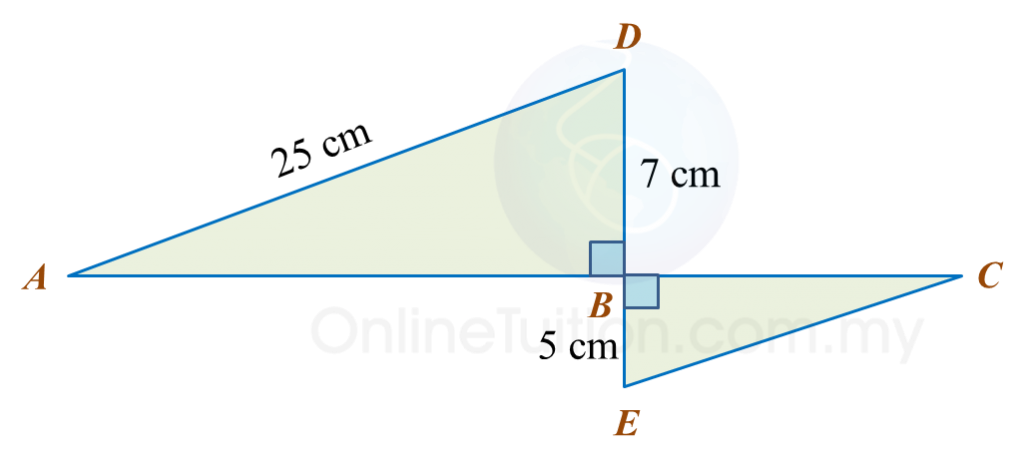Calculate the length, in cm, of CE.

Solution:

Question 9:
Diagram below shows two right-angled triangles, ABE and CBD. BED is a straight line.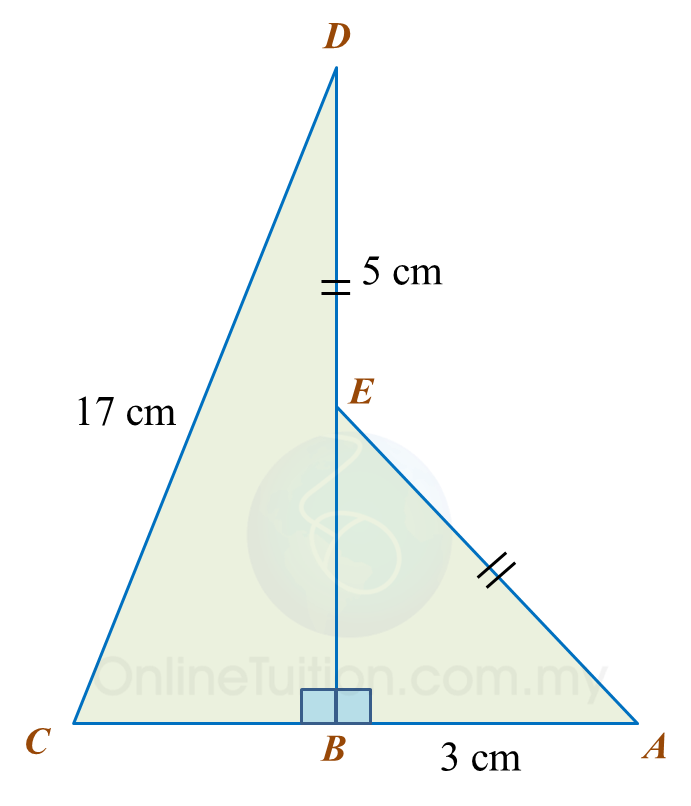Find the length, in cm, of BC. Round off the answer to two decimal places.

Solution:

Question 10:
Diagram below shows two right-angled triangles, ABC and ADE. ACD is a straight line.Find the length, in cm, of AE. Round off the answer to one decimal places.

Solution:

# 6.2.1 Pythagoras’ Theorem, PT3 Focus Practice

6.2.1 Pythagoras’ Theorem, PT3 Focus Practice

Question 1:
In the diagram, ABC is a right-angled triangle and BCD is a straight line. Calculate the length of AD, correct to two decimal places.

Solution:
In ∆ ABC,
BC= 52 – 42
= 25 – 16
= 9
BC = √9
= 3 cm

In ∆ ABD,
BD = BC + CD
= 3 + 6
= 9
= 16 + 81
= 97
= 9.849
= 9.85 cm

Question 2:
In the diagram shows two right-angled triangles. Calculate the perimeter of the whole diagram.

Solution:
In ∆ ABC,
AC= 62 + 82
= 36 + 64
= 100
AC = √100
= 10 cm

In ∆ EDC,
EC= 122 + 52
= 144 + 25
= 169
EC = √169
= 13 cm

Perimeter of the whole diagram
= AB + BC + CE + DE + AD
= 6 + 8 + 13 + 12 + 5
= 44 cm

Question 3:
Diagram below shows a triangle ACD and ABC is a straight line.
Calculate the length, in cm, of AD.

Solution:
In ∆ DBC,
BC= 252 – 242
= 625 – 576
= 49
BC = √49
= 7 cm
AB = 17 – 7 = 10 cm

In ∆ DAB,
= 100 + 576
= 676
= 26 cm

Question 4:
In diagram below ABDE is a square and EDC is a straight line.
The area of the square ABDE is 144 cm2.
Calculate the length, in cm, of BC.

Solution:
BD = √144
= 12 cm
BC= 122 + 92
= 144 + 81
= 225
BC = √225
= 15 cm

Question 5:
In the diagram, ABCD is a trapezium and AED is a right-angled triangle.

Calculate the area, in cm2, of the shaded region.

Solution:
= 25 + 144
= 169
= 13 cm
Area of trapezium ABDC
= ½ (13 + 15) × 9
= 126 cm2

Area of triangle AED
= ½ × 5 × 12
= 30 cm2

= 126 – 30
= 96 cm2

# 6.1 Pythagoras’ Theorem

6.1 Pythagoras’ Theorem

6.1.1 Pythagoras’ Theorem
1. In a right-angled triangle, the hypotenuse is the longest side of the triangle.
2. Pythagoras’ Theorem:

 In a right-angled triangle, the square   of the hypotenuse is equal to the sum   of the squares of the other two sides.
Example 1:

Solution:
$\begin{array}{l}{x}^{2}={5}^{2}+{12}^{2}\\ \text{}=25+144\\ \text{}x=\sqrt{169}\\ \text{}=13\end{array}$

Example 2:

Solution:
$\begin{array}{l}{x}^{2}={15}^{2}-{9}^{2}\\ \text{}=225-81\\ \text{}x=\sqrt{144}\\ \text{}=12\end{array}$

3. Pythagorean triples
are three whole numbers that form the sides of a right-angled triangle.

Example:
(a) 3, 4, 5
(b) 6, 8, 10
(c) 5, 12, 13
(d) 8, 15, 17
(e) 9, 12, 15

6.1.2 The Converse of the Pythagoras’ Theorem

 In a triangle, if the sum of the squares of the two sides   is equal to the square of the longest side, then the angle   opposite the longest side is a right angle.